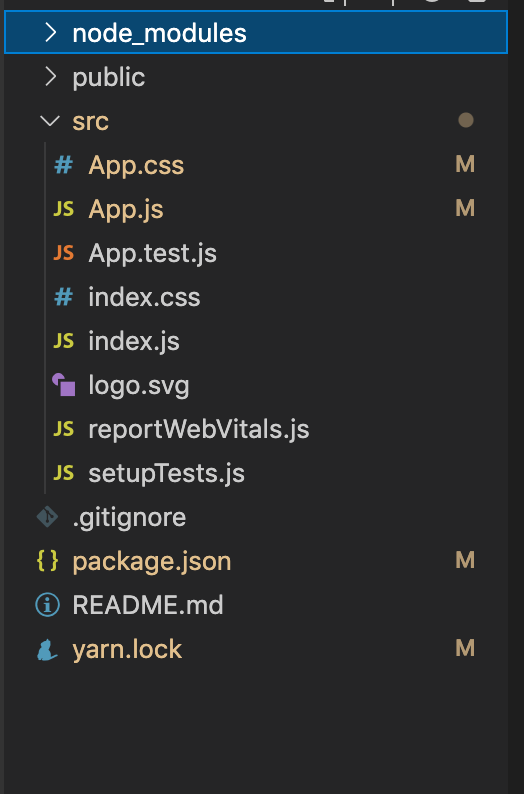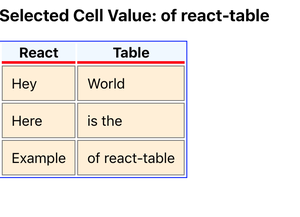# How to get cell value on React-Table ?

• Last Updated : 31 Jul, 2021

React Table is a library that helps us to create a table and gives us many features which we can perform on a table.

Some Features of React Table:

• Lightweight (5kb-14kb+ depending on features used and tree-shaking).
• Headless (100% customizable, Bring-your-own-UI).
• Auto out of the box, fully controllable API.
• Sorting (Multi and Stable)
• Filters.
• Pivoting & Aggregation.
• Virtualizable.
• Resizable

Get Cell Value: We can get the cell/column value of the table by adding the onClick event to the <td> tags.

`getCellValue function : onClick={()=> setCellValue(cell.value)}`

Creating React Application And Installing Module:

Step 1: Create a React application using the following command.

`npx create-react-app foldername`

Step 2: After creating your project folder i.e. foldername, move to it using the following command.

`cd foldername`

Step 3: After creating the ReactJS application, Install the react-table using the following command.

`npm i react-table`

Project Structure: It will look like the following.Project Structure

Example: Now write down the following code in the App.js file. Here, App is our default component where we have written our code.

## Javascript

 `import React, { useState } from ``'react'``;``import { useTable } from ``'react-table'``;`` ` `const  App = () => {` `  ``// Define state``  ``const [cellValue, setCellValue] = useState(``''``);` `  ``// Add data here to show in table``  ``const data = React.useMemo(``    ``() => [``      ``{``        ``reactCol1: ``'Hey'``,``        ``reactCol2: ``'World'``,``      ``},``      ``{``        ``reactCol1: ``'Here'``,``        ``reactCol2: ``'is the'``,``      ``},``      ``{``        ``reactCol1: ``'Example'``,``        ``reactCol2: ``'of react-table'``,``      ``},``    ``],``    ``[]``  ``)` `  ``// Define column of the table``  ``const columns = React.useMemo(``    ``() => [``      ``{``        ``Header: ``'React'``,` `        ``// Accessor is the "key" in the data``        ``accessor: ``'reactCol1'``,``      ``},``      ``{``        ``Header: ``'Table'``,``        ``accessor: ``'reactCol2'``,``      ``},``    ``],``    ``[]``  ``)` `  ``// Function to get cell value``  ``const getCellValue = (cell) =>{``    ``setCellValue(cell.value)``  ``}` `  ``// Create the instance of table by``  ``// using hooks of react-table``  ``const {``    ``getTableProps,``    ``getTableBodyProps,``    ``headerGroups,``    ``rows,``    ``prepareRow,``  ``} = useTable({ columns, data })` `  ``return` `(``    ``<>``    ``{``/* Showing cell value */``}``    ``

Selected Cell Value: {cellValue}

``    ``` `      ``
``        ``{headerGroups.map(headerGroup => (``          ````            ``{headerGroup.headers.map(column => (``              ````                ``{column.render(``'Header'``)}``              ````            ``))}``          ````        ``))}``      ````      ``
``        ``{rows.map(row => {``          ``prepareRow(row)``          ``return` `(``            ````              ``{row.cells.map(cell => {``                ``return` `(``                  ``<>``                  ``{``/* Here added onClick function to get cell value */``}``                  `` getCellValue(cell)}``                    ``{...cell.getCellProps()}``                    ``style={{``                      ``padding: ``'10px'``,``                      ``border: ``'solid 1px gray'``,``                      ``background: ``'papayawhip'``,``                    ``}}``                  ``>``                    ``{cell.render(``'Cell'``)}``                  ````                  ````                ``)``              ``})}``            ````          ``)``        ``})}``      ````    ````    ````  ``)``}` `export ``default` `App;`

Step to Run Application: Run the application using the following command from the root directory of the project.

`npm start`

Output: Now open your browser and go to http://localhost:3000/, you will see the following output by clicking the of react-table cellOutput

My Personal Notes arrow_drop_up